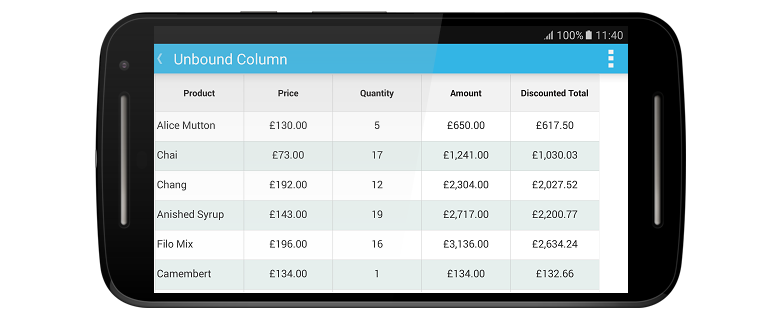# Unbound Column in Xamarin.Android SfDataGrid

30 Jun 20224 minutes to read

The dataGrid adds additional columns which are not bound with data object from the underlying data source. Add unbound column using SfDataGrid.GridUnboundColumn class.

• C#
• ``````SfDataGrid dataGrid = new SfDataGrid(context);
UnBoundColumnViewModel viewModel = new UnBoundColumnViewModel();
dataGrid.ItemsSource = viewModel.Products;

GridUnboundColumn AmountColumn = new GridUnboundColumn();
AmountColumn.MappingName = "Amount";
AmountColumn.Expression = "UnitPrice * Quantity";
AmountColumn.Format = "C";
AmountColumn.TextAlignment = GravityFlags.Center;

GridUnboundColumn TotalColumn = new GridUnboundColumn();
TotalColumn.MappingName = "Total";
TotalColumn.Expression = "(Quantity * UnitPrice) - ((Quantity * UnitPrice)/100 * Quantity)";
TotalColumn.Format = "C";
TotalColumn.TextAlignment = GravityFlags.Center;NOTE

It is mandatory to specify the GridColumn.MappingName for `SfDataGrid.GridUnboundColumn` with some name to identify the column. It is not necessary to define the name of the field in the data object.

## Populating data for unbound column

You can populate the data for unbound column by setting Expression or Format property.

### Using Expression

Specifies the arithmetic or logic expression using `Expression` property to compute the display value. By default, `GridUnboundColumn` evaluates the expression with casing. Disable the casing while evaluate the expression by setting the CaseSensitive property to `false`.

Following list of arithmetic and logical operations supported:

Arithmetic operations Operator
Subtract -
Multiply *
Divide /
Power ^
Mod %
Greater than >
Less than <
Equal =
GreaterThanOrEqual >=
LessThanOrEqual <=

Logical Operations

Logical operations Operators
AND (char)135
OR (char)136
NOT (char)137
• C#
• ``````SfDataGrid dataGrid = new SfDataGrid(context);
UnBoundColumnViewModel viewModel = new UnBoundColumnViewModel();
dataGrid.ItemsSource = viewModel.Products;

GridUnboundColumn TotalColumn = new GridUnboundColumn();
TotalColumn.MappingName = "Total";
TotalColumn.Expression = "(Quantity * UnitPrice) - ((Quantity * UnitPrice)/100 * Quantity)";

### Using Format

Format the value of other columns and display the formatted value in the unbound column using `Format` property.

• C#
• ``````SfDataGrid dataGrid = new SfDataGrid(context);
UnBoundColumnViewModel viewModel = new UnBoundColumnViewModel();
dataGrid.ItemsSource = viewModel.Products;

GridUnboundColumn TotalColumn = new GridUnboundColumn();
TotalColumn.MappingName = "Total";
TotalColumn.Format = "C";

### Using QueryUnboundColumnValue event

The QueryUnboundColumnValue event is fired when value for the unbound column is queried. It provides information about the cell triggered this event so, you can set the desired value for the grid cells of the unbound column. This event is triggered with GridUnboundColumnEventsArgs.

The `GridUnboundColumnEventsArgs` provides the following properties:

• Column: Gets the `GridColumn` of the cell that triggers this event.
• OriginalSender: Gets the data grid raising this event.
• Record: Gets the underlying row data.
• UnboundAction: Defines the action for triggering this event.
• Value: Gets or sets the value for `GridUnboundColumn` cell based on `UnboundAction`.

NOTE

`UnboundActions.CommitData` and `UnboundActions.PasteData` are currently not supported and likely to be supported in future.

You can populate data for the unbound column by handling `QueryUnboundColumnValue` event to customize the value of the `GridUnboundColumn`. `GridUnboundColumnEventsArgs` exposes the `Value` property by which you can set the value for the grid cells of the unbound column based on the `UnboundAction`.

Refer to the following code example in which data for the unbound column is populated by handling the `QueryUnboundColumnValue` event.

• C#
• ``````dataGrid.QueryUnboundColumnValue += DataGrid_QueryUnboundColumnValue;

private void DataGrid_QueryUnboundColumnValue(object sender, GridUnboundColumnEventsArgs e)
{
if (e.UnboundAction == UnboundActions.QueryData)
{
var quantity = Convert.ToInt16(e.Record.GetType().GetProperty("Quantity").GetValue(e.Record));
var unitPrice = Convert.ToInt16(e.Record.GetType().GetProperty("UnitPrice").GetValue(e.Record));
var amount = quantity * unitPrice;
e.Value = amount;
}
}``````